# Excel Formula Not WorkingArticle byExcelMojo Team## What Is Excel Formula Not Working?

An Excel formula not working is a type of Excel formula error. This issue occurs when the formula returns an incorrect result or an error value instead of the calculated results.

Typically, users face an Excel formula not working when they enter it with syntax or input values’ format issues or incorrect arguments. The problem might also occur when working with multiple workbooks containing a large amount of data and formulas.

For example, the below image shows two tables. The first table contains the subject scores of 10 students.

Select cell H2, and enter the VLOOKUP() formula =VLOOKUP(G2,A:C,3,0,FALSE) to display the Physics score of the student specified in cell G2 in the second table.

• Error/Warning → We get the error that we entered too many arguments.
• Reason → The entered VLOOKUP excel function contains one additional argument.
• Correct formula=VLOOKUP(G2,A:C,3,FALSE)         Or            =VLOOKUP(G2,A:C,3,0)
• Right Output: We will get the correct Physics score of the specified student, Lisa Maxwell, 98.
• Error Observation and Solution: Thus, we can avoid such a case of the Excel formula not working warning by checking if the entered formula or function contains all the required arguments.
###### Key Takeaways
• The Excel formula not working is an Excel formula error condition, where the specific formula returns a warning/error message or an incorrect value.
• Users might find Excel formula not working when their worksheets contain massive datasets involving formulas. They might also face the issue when referring to multiple workbooks and worksheets in the active spreadsheet.
• If the Excel formulas do not update automatically, check the Calculation Options setting in the Formulas tab. It should be Automatic.
• If we find the Excel formulas not calculating, check for the following causes:
• Show Formulas mode is On.
• The entered formula is in the Text format.
• The entered formula contains a leading space or apostrophe before the ‘=’ sign.

### Top 10 Reasons (with Solutions)

We will consider the Top 10 reasons for the Excel formula not working and the solutions.

#### #1 – Mismatch in Opening and Closing Parentheses in a Function/Formula

Typically, complex Excel formulas include multiple sets of opening and closing parentheses. They help define the order of the calculations within the formula.

However, if we enter an opening parenthesis but miss its matching closing parenthesis or vice versa in a formula, we may have the scenario of the Excel formula not working.

For example, the first table in the image below shows a list of items and their order details.

To determine the order status for the item specified in the second table based on the units required and ordered data in the first table, select cell F2, and enter the formula =IF((VLOOKUP(E2,A:C,3,0)>VLOOKUP(E2,A:B,2,0)),”Order Fulfilled”,”Order Unfulfilled” as shown below.

However, on applying the formula, we get the above warning message. The reason is the opening and closing parentheses mismatch. Excel identifies it as a typo error and suggests correcting the issue. In this case, the formula is missing the last closing parenthesis. So, we can click Yes in the error message window or close the window and manually enter the last closing parenthesis to get the correct result.

Solution

We can check the following points to avoid such a condition of the Excel formula not working.

• Excel highlights each opening and closing parenthesis pair in a different color. We can check the colors to locate the missing or additional parenthesis.
• While entering the closing parenthesis, Excel will momentarily make its opening parenthesis pair bold. And if it does not show the matching opening parenthesis in bold, it indicates a mismatch.
• When we click the Formula Bar or double-click the cell containing the formula, Excel shows the opening and closing parenthesis pair in the respective colors. We can use the color code to check for the missing parenthesis.

#### #2 – Function Arguments not Seperated with the Required Character

We might have an Excel formula not working if we do not use the required list separator in the formula properly.

For example, once again, consider the previous example. If we enter the formula as depicted in the image below, we will have the condition of the Excel formula not working.

The IF excel function is missing the list separator, ‘,’ between the TRUE and FALSE values.

However, if we do not use a list separator according to our Excel regional settings, our Excel formulas might also not work in such a scenario.

For example, the formula, =IF((VLOOKUP(E2,A:C,3,0)>VLOOKUP(E2,A:B,2,0)),”Order Fulfilled”,”Order Unfulfilled”) might not work in European countries, as they consider a ‘;’ as a list separator, while the ‘,’ works in North America.

Solution

• Confirm whether we included the list separator at every required position in the formula before executing it.

For example, in the above IF(), once we enter the list separator, ‘,’, between the TRUE and FALSE values, the function will return the correct result.

• Go to Control PanelRegion and LanguageAdditional Settings to check the Regional Settings and the accepted List Separator character. And then ensure we use the applicable list separator in our Excel formulas.

#### #3 – All Required Function Arguments Not Provided

Typically, an Excel function requires mandatory arguments. However, some also include optional arguments for which the functions take the default values if not provided.

But when we provide only some of the mandatory arguments to the specific formula, we will have the scenario of the Excel formula not working.

For example, the following table contains the mandatory arguments required to compute the compound interest in cell B8.

But when we enter the FV excel function in the target cell B8 to get the compound interest, as depicted in the image below, we will get the Excel formula not working.

The warning message says the entered arguments are too few for this function. And if we enter more than the required arguments, Excel returns a warning message for such issues, stating the entered arguments are too many for this function.

Solution

We can enter the Excel formula in two ways, which will help us avoid this situation.

• Enter the formula directly in the target cell. Once we enter the ‘=’ symbol and the function name, Excel will show the mandatory and optional arguments we need to provide to execute it. For example, in the above table, we will see the FV formula syntax.

Excel will highlight the argument to enter.

And finally, when we close the parenthesis and press Enter, the formula will get executed.

• We can use the function from the Formulas tab, which allows us to enter the arguments in the Function Arguments window. It will ensure that we enter all the required arguments. For example, the FV() is a Financial formula in the Formulas tab. So, we can select the target cell and navigate as depicted below to enter the arguments in the Function Arguments window.

The Function Arguments window opens, showing the mandatory argument names in bold.

Enter the mandatory arguments correctly in the window and click OK, as shown below.

The formula executed gives the following output.

#### #4 – Incorrect Function Syntax

When we enter a function with incorrect syntax, it can lead to the Excel formula not working.

For example, if we enter the function name incorrectly in the previous example, the formula will not work.

The entered function is FB() instead of FV(). Thus, the function returns the #NAME? error.

Likewise, the last optional argument in the FV() can take a value of 0 or 1. Or we can enter the argument value as FALSE or TRUE without double quotes. And if we enter the last argument, Type, as a value other than the mentioned options, it will result in the Excel formula not working.

In the above example, the last argument value is in double quotes, leading to the function returning the #VALUE! error.

Solution

• Ensure we enter the correct Excel function or the required formula.
• Check the function or formula syntax for correct argument values before executing the function.

For example, we will get the required output when correctly entering the last argument value without the double quotes in the above FV().

#### #5 – A Number Value in an Excel Formula is in Double Quotations or Text Format

When we enter a number value in a formula but within double quotes or in the text format, it leads to the Excel formula not working.

For example, the first table contains a list of values. And the second table shows two conditions to get the required results.

Select cell E2, and enter the IF() formula to achieve the desired outcome.

The cell E2 shows the correct result, 1, as the sum of all the values in the first table is 15, a value greater than 4. Unfortunately, Excel treats the function return value as a text string. And thus, we cannot use it in other Excel functions to perform calculations.

On the other hand, if the provided values in the first table are in text format. And we use them in an Excel function to perform the required calculations. Then, it will lead to the Excel function not working.

For example, the first table values are in Text format, as the Number Format in the Home tab shows the cells’ data format as Text.

Now, if we use the first table values in the SUM excel function in the cell E3, the output will be:

While the correct result is 15, since all the number values in the first table are in text format, the SUM() considers them as text values and thus returns an incorrect output, 0.

Also, in such conditions, the Status Bar showing only the function COUNT indicates that the worksheet contains many number values in text format. Otherwise, we should be able to see other Excel functions, such as SUM and AVERAGE.

Solution

• Ensure we do not provide number values in double quotations to an Excel function.
• If we see number values left-aligned in cells, check the Number Format option in the Home tab and change the format from Text to General or Number.

However, if the issue persists even after changing the Number Format, delete the data and enter the values again with the Number Format being General or Number.

• We can select all the error cells, click the error message and choose the option Convert to Number.

And once we choose the option mentioned above, the SUM() in cell E3 will return the correct value.

#### #6 – Number Values Entered with Formatting

When we enter numbers with formatting such as Currency while typing a formula, we may end up with the Excel formula not working.

For example, the below table shows a list of items, their prices per unit, and the number of units ordered.

To find the total value of the order in \$ for each item to display the result in column D, we will apply the above-shown formulas in column D, with a ‘\$’ symbol before the column B values, as we want to display the result as currency values. However, the resulting amounts in column D are not currency values.

Solution

• Avoid using a decimal separator or currency sign, such as ‘\$’, while entering a number in an Excel function to avoid the scenario of the Excel formula not working. The reason is, typically, commas separate function arguments, and the ‘\$’ symbol before a cell reference makes it an absolute cell reference.
• After entering the number as a plain number, right-click the cell and choose the Format Cells option from the contextual menu to apply the required formatting.

For example, remove the ‘\$’ symbol in the column D formulas in the above table.

Then, use the Format Cells option, as explained above, to get the required result.

The “Format Cells” window appears.

The “Number Format” is now changed to “Currency”.

#### #7 – Using Absolute Reference Incorrectly

When we do not properly use absolute references in a function, it might lead to the Excel formula not working.

For example, the first table shows a list of students, their admission numbers, and grade details.

The second table contains the same list of students but in alphabetical order, and we have to update their grades using the data in the first table. So, we will apply the VLOOKUP() in the target cells without using the absolute reference for the lookup range to get the following output.

The issue is the lookup range in the VLOOKUP() has to be the same in all the target cells. But, as the VLOOKUP() applied in the first target cell does not use the absolute reference to the lookup range, the range changes when we copy the VLOOKUP() in the following cells. It thus leads to the Excel formula not working in each cell that falls outside the lookup range.

Solution

• Make the required cell reference absolute in the formulas to use the same cell value in the specific target cells’ functions.

For example, once we provide the absolute cell reference to the lookup range in the above VLOOKUP functions, the range remains the same in all the target cells. And the output will be:

#### #8 – Nesting More Than 64 Functions in an Excel Formula

When applying Excel formulas such as the Nested IF function, we might nest more than 64 functions in the formula to meet our requirement. However, such a scenario will lead to the Excel formula not working.

The reason is that the maximum nesting capacity in Excel version 2007 and above is 64. On the other hand, the function nesting capacity in Excel versions before 2007 is 7.

Solution

• If our Excel version is 2007 or above, ensure we use up to 64 levels of nested functions.
• If our Excel version is before 2007, we can have the maximum nested function level of 7.

#### #9 – Workbook or Worksheet Names in a Formula Not Enclosed in Single Quotations

To refer to other worksheets or workbooks in our formula, but their names contain spaces or non-alphabetic characters, we must enclose their names in single quotations to avoid the scenario of the Excel formula not working.

The below image shows two tables. And is we need to update the first and second tables using data from another workbook and a worksheet in the current worksheet.

1. Select cell A2, and enter the formula =SUM([LOOKUP Excel Function_Worksheet.xlsx]LOOKUP_FAQ!\$B\$2:\$B\$9)

[Note: The workbook name contains spaces, and as we did not enclose the name in single quotations, we see the above warning message.]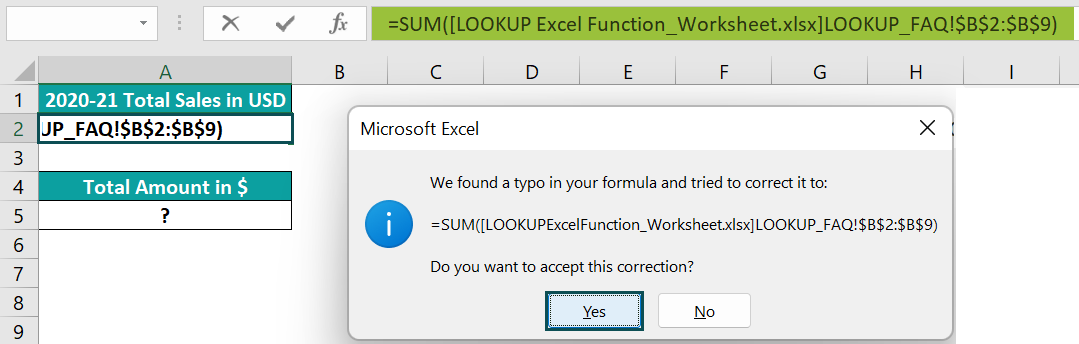2. Select cell A5, and enter the formula =SUM(EFNW ‘6′!D2:D5)

[Note: The single quotations do not enclose the entire worksheet name containing a space. And thus, Excel does not recognize the worksheet name and returns the #NAME? error.]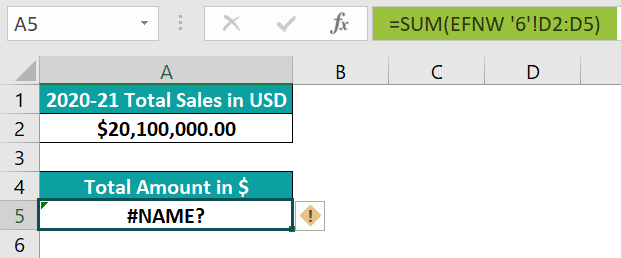Solution

• Enter the formula with the workbook name in single quotations to get the required data,

as =SUM(‘[LOOKUP Excel Function_Worksheet.xlsx]LOOKUP_FAQ’!\$B\$2:\$B\$9)

• Enclose the entire worksheet name in single quotations to achieve the desired results,

as =SUM(‘EFNW 6’!D2:D5)

#### #10 – Full Path of Closed Workbook Not Provided while Referring to It in the Formula

When referring to another closed workbook in a formula, the external reference should include the entire path to the workbook.

For example, cell A2 contains a formula that refers to a closed workbook. And if we do not provide the entire path to the workbook, it might lead to the Excel formula not working.

Solution

• When referencing a closed workbook in a formula, ensure we include the entire path to the workbook and its name in the external reference.

### Why Excel Formulas Not Updating?

Sometimes, the formula does not return the updated value automatically. Instead, it shows the previous result, even after updating the required cell references.

For example, the table below shows the monthly savings calculations in column D.

However, even when the formulas in cells D3:D7 contain the respective dependent cells’ references, the output in every cell in column D remains the same, cell D2 result.

The reason is that the Calculation Options setting is not Automatic. Instead, it is Manual.

Solution

• Set the Calculation settings as Automatic

Go to the File tab.

Choose the Options option.

Next, click the Formulas tab in the menu and set the Calculation options as Automatic.

The target cells will automatically get updated with the correct function return values.

• If we want the Calculation Options to be Manual and to recalculate the entire Excel workbook, we can force the calculation using the shortcut keys F9 or FormulasCalculate Now.

And if we need to recalculate an active worksheet, press the shortcut keys Shift + F9 or use FormulasCalculate Sheet.

On the other hand, by pressing Ctrl + Alt + F9, we can recalculate all the worksheets in all the open workbooks. But if we need to recalculate a single formula in a worksheet, select the specific cell with the formula and enter the edit mode. We can do so by pressing the F2 key or double-clicking the specific cell. And finally, press Enter to execute the formula.

### Why Excel Formulas Not Calculating?

When we enter a formula in a cell, the cell displays the formula instead of the formula’s calculated value. Then, check for the below issues to ensure the formula works.

1. Show Formulas Mode Is On.
2. The Formula Entered as A Text.
3. The Target Cell Formula Has A Leading Space or Apostrophe Before The ‘=’ Sign.

Let us understand in detail and look into the workaround.

#### #1 – Show Formula Mode is On

When we enter the required formulas in column B in the table below, the target cells show the entered formulas instead of their return values.

The reason is the Show Formulas mode in the Formulas tab is ON.

Solution

• Ensure the Show Formulas mode is OFF to view the formula return values. And for that, we can press Ctrl + ` or unselect the Show Formulas option in the Formulas tab.

#### #2 – The Formula Entered as a Text

Considering the previous example, if the target cells display the entered formulas instead of their return values, we must check the target cells’ Number Format in the Home tab. If it is Text, it could be the reason for this issue.

Solution

• Set the target cell’s Number Format in the Home tab as General.

The “Number Format” is now in “General” format.

And then, select cell B2 and press F2 to go to the edit mode.

Next, press Enter to recalculate the formula and get its return value.

Finally, repeat the above steps to recalculate the formulas in cells D3:D6 or use the fill handle to copy the cell D2 formula in cells D3:D6.

#### #3 – The Target cell Formula Has a Leading Space or Apostrophe Before the ‘=’ Sign

Continuing with the previous example, if we enter the required formula in cell B2 but with a leading space or an apostrophe in front of the ‘=’ sign, then we will see the formula in the target cell and not its evaluated value, as shown in the images below.

Solution

• When entering a formula in the target cell, ensure we do not enter a leading space or an apostrophe before the ‘=’ sign. And when copying a formula from the web, ensure there is no space before the ‘=’ sign.

### Important Things to Note

• While applying the formula in a cell, ensure the function syntax is correct, with the correct number of matching parentheses and appropriate list separators. Also, check if we have entered the values for all the mandatory arguments to the function. Otherwise, it might lead to the Excel formula not working.
• Do not enter numbers in the formulas as text values or within double quotations.
• Use absolute references properly, and do not nest more than 64 functions in a formula.

1. What are the different types of Excel formula errors?

Some of the Excel formula errors are as follows:
The Excel formula is not working.
The Excel formula is not updating.
Excel formula not calculating.
Too many arguments entered in the formula or too few arguments without the mandatory argument values.

2. Can an incorrect cell format lead to the Excel formula not working?

An incorrect cell format can lead to the Excel formula not working, specifically if it’s a Text format.

For example, the table below shows the length and breadth values of a rectangle. And column C contains the formulas for calculating the perimeter of the rectangle based on the respective length and breadth values.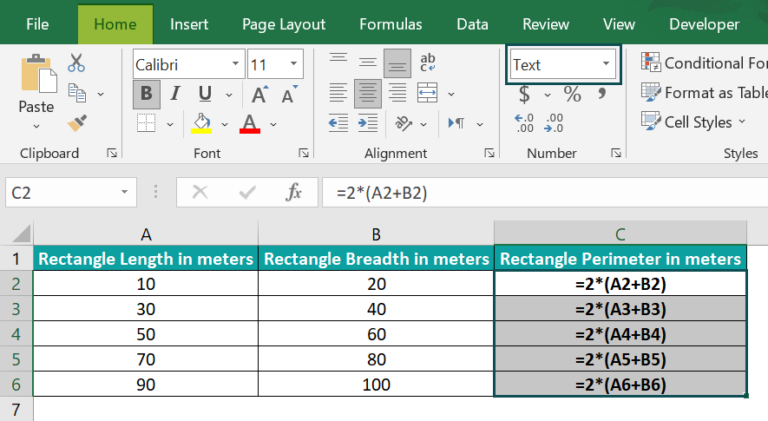However, the target cells show the formulas instead of the formula values. The reason is that the target cells’ format is Text, as highlighted in the above image.
The solution is as follows:

• 1: First, select the target cells, C2:C6, and update the Number Format option in the Home tab as General.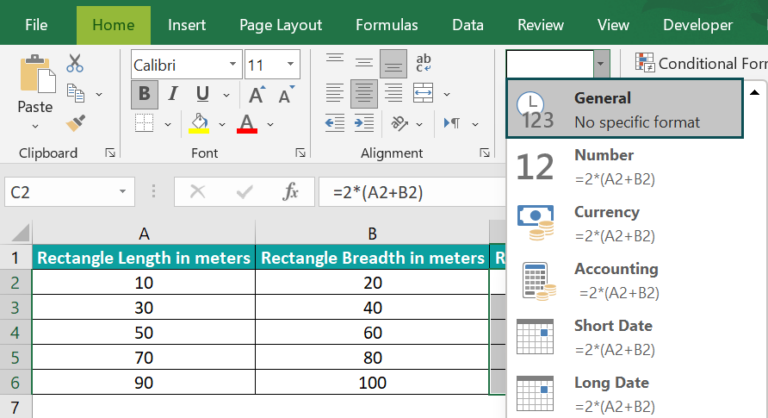The “Number Format” is now in the “General” format.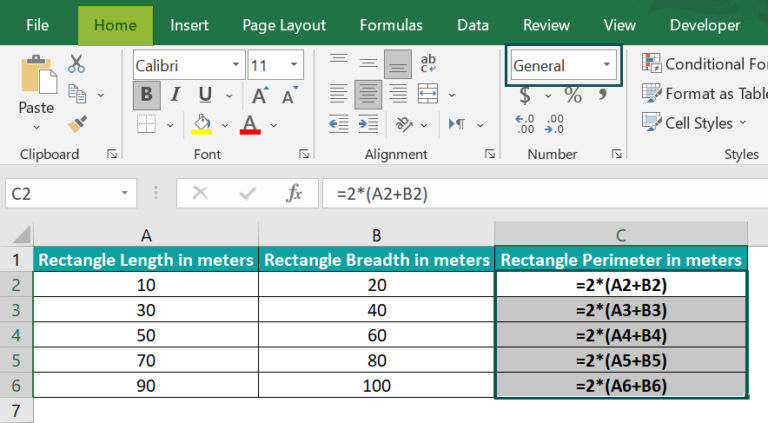2: Double-click cell C2 to go to the edit mode.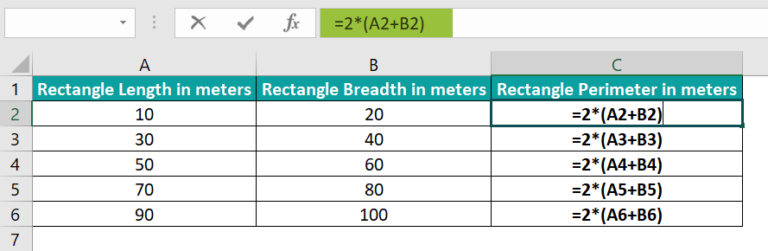And press Enter.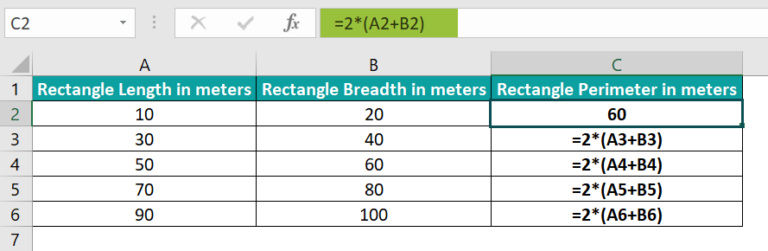• 3: Drag the fill handle downwards to copy the formula in cells C3:C6.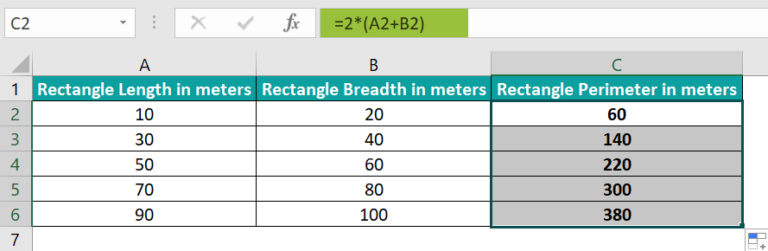3. What happens when we enclose a worksheet name while referring to it in a formula in our active worksheet in double quotes?

When we enclose a worksheet name while referring to it in a formula in our active worksheet in double quotes, we will get the below error.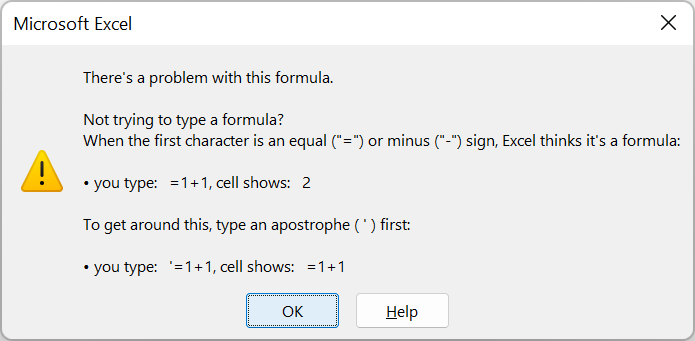The solution to this issue is to remove the double quotations and enclose the worksheet name in single quotes if the name contains space. Otherwise, we can enter the worksheet name without quotes.